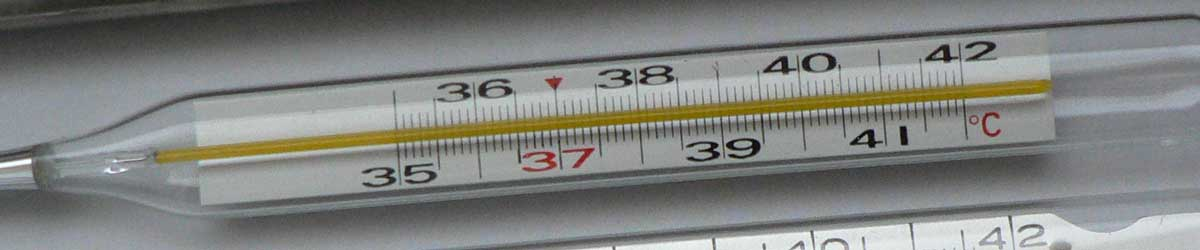# Newton to Celsius Conversion

Enter the temperature in Newton below to get the value converted to Celsius.

Results in Degrees Celsius:1 °N = 3.030303 °C
Do you want to convert Celsius to Newton?

## How to Convert Newton to CelsiusConvert degrees Newton to degrees Celsius with this simple formula:

degrees Celsius = [°N] × 100 / 33

Insert the [°N] temperature measurement in the formula and then solve to find the result.

For example, let's convert 50 °N to degrees Celsius:

50 °N = ( 50 × 100 / 33 ) = 151.515152 °C

## Newton

The Newton scale is a temperature scale that sets a fixed zero point equal to the freezing point water. The scale uses both arithmetic progression and geometric progression. The scale defines 18 reference points and the corresponding "degrees of heat" and geometric progressions.

Newton can be abbreviated as N; for example, 1 degree Newton can be written as 1 °N.

## Celsius

The Celsius temperature scale, also commonly referred to as the centigrade scale, is defined in relation to the kelvin. Specifically, degrees Celsius is equal to kelvins minus 273.15.

The degree Celsius is the SI derived unit for temperature in the metric system. A degree Celsius is sometimes also referred to as a degree centigrade. Celsius can be abbreviated as C; for example, 1 degree Celsius can be written as 1 °C.

### Important Temperatures In Degrees Celsius

TemperatureDegrees Celsius
Absolute Zero-273.15 °C
Freezing Point of Water0 °C
Triple Point of Water0.01 °C
Boiling Point of Water100 °C

## Newton to Celsius Conversion Table

Newton measurements converted to Celsius
Newton Celsius
-50 °N -151.52 °C
-40 °N -121.21 °C
-30 °N -90.91 °C
-20 °N -60.61 °C
-10 °N -30.3 °C
0 °N 0 °C
10 °N 30.3 °C
20 °N 60.61 °C
30 °N 90.91 °C
40 °N 121.21 °C
50 °N 151.52 °C
60 °N 181.82 °C
70 °N 212.12 °C
80 °N 242.42 °C
90 °N 272.73 °C
100 °N 303.03 °C
110 °N 333.33 °C
120 °N 363.64 °C
130 °N 393.94 °C
140 °N 424.24 °C
150 °N 454.55 °C
160 °N 484.85 °C
170 °N 515.15 °C
180 °N 545.45 °C
190 °N 575.76 °C
200 °N 606.06 °C
300 °N 909.09 °C
400 °N 1,212 °C
500 °N 1,515 °C
600 °N 1,818 °C
700 °N 2,121 °C
800 °N 2,424 °C
900 °N 2,727 °C
1,000 °N 3,030 °C

## References

1. National Institute of Standards and Technology, SI Units - Temperature, https://www.nist.gov/pml/weights-and-measures/si-units-temperature